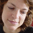# Processing.js

## Brightness

by Daniel Shiffman. Adjusts the brightness of part of the image Pixels closer to the mouse will appear brighter.

Original Processing.org Example: Brightness```// All Examples Written by Casey Reas and Ben Fry
// unless otherwise stated.
PImage img;

void setup() {
size(200, 200);
frameRate(30);
}

void draw() {
for (int x = 0; x < img.width; x++) {
for (int y = 0; y < img.height; y++ ) {
// Calculate the 1D location from a 2D grid
int loc = x + y*img.width;
// Get the R,G,B values from image
float r,g,b;
r = red (img.pixels[loc]);
//g = green (img.pixels[loc]);
//b = blue (img.pixels[loc]);
// Calculate an amount to change brightness based on proximity to the mouse
float maxdist = 50;//dist(0,0,width,height);
float d = dist(x,y,mouseX,mouseY);